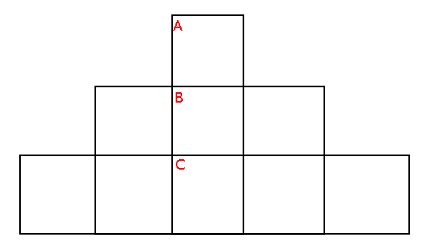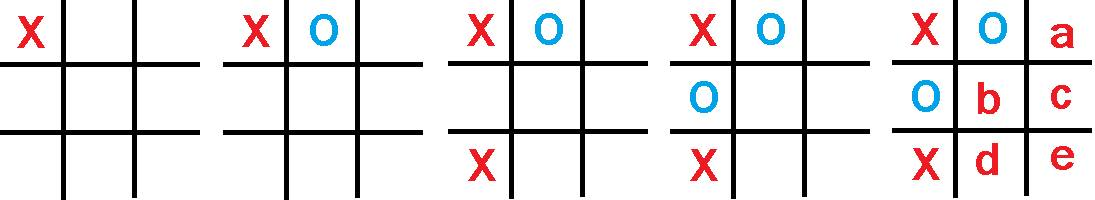Probability

# Combinatorial Games: Level 3 Challenges

An intelligent trader travels from 1 place to another carrying 3 sacks having 30 coconuts in each of them. No sack can hold more than 30 coconuts and he can move the coconuts between sacks. On the way, he passes through 30 checkpoints and on each checkpoint he has to give 1 coconut from each sack he is carrying.

What is the maximum number of coconuts he can have after passing through every checkpoint?Alice and Bob are playing Tic-Tac-Toe on the above triangular board. They play as in in normal Tic-Tac-Toe: taking turns, each player claims one square. The first to claim three squares in a row (vertically, horizontally, or diagonally) wins.

Alice plays first. Alice and Bob both want to win and play optimally. Which of the following is true?

Written on a blackboard is the polynomial ${ x }^{ 2 }+x+2014$ Calvin and Hobbes take turns alternatively (starting with Calvin) in the following game. During his turn, Calvin should either increase or decrease the coefficient of x by 1. And during his turn, Hobbes should either increase or decrease the constant coefficient by 1. If Calvin wins if at any point of time the polynomial on the blackboard at that instant has integer roots, then Who Has A Winning Strategy?

Alice and Carla are playing a game often learned in elementary school known as Twenty One. The rules for the game are as follows:

• Each player takes turns saying between $1$ and $3$ consecutive numbers, with the first player starting with the number $1$. For example, Player $1$ could say the numbers $1$ and $2$, then Player $2$ can say "$3$, $4$, $5$", then Player $1$ can say "$6$" and so on.

• The goal of the game is to get the other person to say "$21$", meaning that you have to be the one to say "$20$".

Carla begins to get bored with the game, so she decides to make it a little bit more challenging. The name of the new game is Two Hundred Thirty Nine, where the goal is now to get the other person to say "$239$". Carla decides that she'll go first and that Alice will go second. Also, each player is now able to say up to "$6$" numbers per turn. Is there a way to tell which player is going to win before the game even starts?

Details and Assumptions:

• Assume that each player plays "perfectly", meaning that if there was an optimal way of playing, both players would be playing the best that the game allows them to play.

Assume that you are playing a tic-tac-toe game with an opponent. You play as $X$ while your opponent plays as $O$.

The game is played as follows:Find all the possible grid tiles where you can put an $X$-mark that will help you guarantee a win under best play.

×